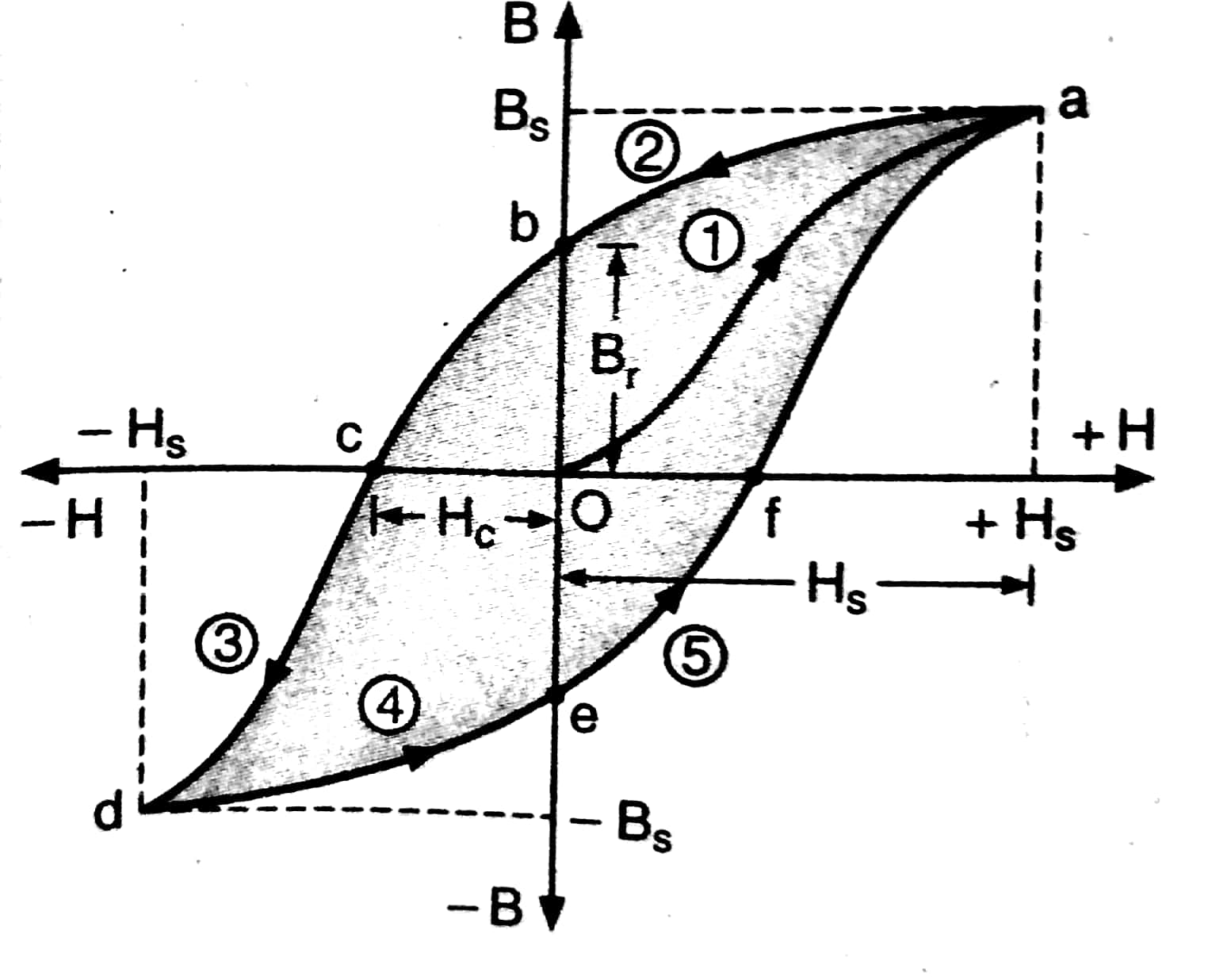## Filters

Sort by :
Clear All
Q
Engineering
504 Views   |

The B-H curve for a ferromagnet is shown
in the figure. The ferromagnet is placed
inside a long solenoid with 1000 turns/cm.
The current that should be passed in the
solenoid to demagnetise the ferromagnet
completely is :• Option 1)

1 mA

• Option 2)

2 mA

• Option 3)

20 μA

• Option 4)

40 μA

As we learned

Hysteresis Curve -

The graph between B and H is called hysteresis curve

- whereinCoercivity (H)= 100

H=nI =100

Option 1)

1 mA

Option 2)

2 mA

Option 3)

20 μA

Option 4)

40 μA

View More
Engineering
519 Views   |

A Helmholtz coil has a pair of loops, each with N turns and radius R. They are placed coaxially at distance R and the same
current I flows through the loops in the same direction. The magnitude of magnetic field at P, midway between the centres A and C, is given by [Refer to figure given below] :• Option 1)

• Option 2)

• Option 3)

• Option 4)

As we learned   Hysteresis Curve - The graph between B and H is called hysteresis curve - wherein     Option 1)       Option 2) Option 3) Option 4)
Engineering
812 Views   |

At the centre of a fixed large circular coil of radius R, a much smaller circular coil of radius r is placed. The two coils are concentric and are in the same plane. The larger coil carries a current I. The smaller coil is set to rotate with a constant angular velocity ω about an axis along their common diameter. Calculate the emf induced in the smaller coil after a time t of its start of rotation.

• Option 1)

• Option 2)

• Option 3)

• Option 4)

As we learnt Magnetic field due to cCrcular Current Carrying arc - - wherein        Magnetic field due to cCrcular Current Carrying arc - - wherein       At time the samller coil is rotated by  Angle between    Option 1) Option 2) Option 3) Option 4)
Engineering
80 Views   |

A current of 1 A is flowing on the sides of an equilateral triangle of side 4.5 × m. The magnetic field at the centre of the triangle will be :

• Option 1)

×

• Option 2)

Zero

• Option 3)

×

• Option 4)

×

As we learnt Magnetic Field Due to a Straight Wire - - wherein                                                                         Total Magnetic field                                             Option 1) ×  Option 2) Zero Option 3)  ×  Option 4) ×
Engineering
895 Views   |

A charge q is spread uniformly over an insulated loop of radius r. If it is rotated
with an angular velocity ω with respect to normal axis then the magnetic moment of
the loop is :

• Option 1)

• Option 2)

• Option 3)

• Option 4)

As we have learned Magnetic moment (M) - M=NiA - wherein N-number of turns in the coil  i-current throughout the coil  A-area of the coil    for any charge distribution              or                     Option 1) This is incorrect Option 2) This is incorrect Option 3) This is incorrect Option 4) This is incorrect
Engineering
192 Views   |

An electron, a proton and an alpha particle having the same kinetic energy are moving in circular orbits of radii re, rp, ra respectively in a uniform magnetic field B. The relation between re, rp, ra is :

• Option 1)

re < ra < rp

• Option 2)

re > rp = ra

• Option 3)

re < rp = ra

• Option 4)

re < rp < ra

Radius of charged particle - -     Option 1) re < ra < rp   This is incorrect Option 2)  re > rp = ra   This is incorrect Option 3) re < rp = ra   This is correct Option 4) re < rp < ra This is incorrect
Engineering
109 Views   |

The dipole moment of a circular loop carrying a current I, is m and the magnetic field at the centre of the loop is B1. When the dipole moment is doubled by keeping the current constant, the magnetic field at the centre of the loop is B2. The ratio  is:

• Option 1)

• Option 2)

2

• Option 3)

• Option 4)

As we learnt that

Magnetic moment (M) -

M=NiA

- wherein

N-number of turns in the coil

i-current throughout the coil

A-area of the coil

Option 1)

This is incorrect

Option 2)

2

This is incorrect

Option 3)

This is incorrect

Option 4)

This is correct

View More
Exams
Articles
Questions